# Common Core Math-Aligned Curriculum

Hands-on engineering curricula aligned to Math Standards encourages students to hone their abstract, spatial, reasoning and critical thinking skills through exploring real-world challenges

Hands-on, Mathematics-Aligned Engineering Curriculum

The TeachEngineering mathematics-aligned activities featured here, by grade, support the Common Core Standards for Mathematics.

• As We Grow: Measuring Heights and Graphing Data
As We Grow: Measuring Heights and Graphing Data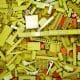Students visit second- and fourth-grade classes to measure the heights of older students using large building blocks as a non-standard unit of measure. They also measure adults in the school community. Results are displayed in age-appropriate bar graphs (paper cut-outs of miniature building blocks g...

• How High Can a Super Ball Bounce?
How High Can a Super Ball Bounce?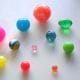Students determine the coefficient of restitution (or the elasticity) for super balls. Working in pairs, they drop balls from a meter height and determine how high they bounce. They measure, record and repeat the process to gather data to calculate average bounce heights and coefficients of elastici...

• Do Different Colors Absorb Heat Better?
Do Different Colors Absorb Heat Better?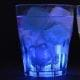Students test whether the color of a material affects how much heat it absorbs. They leave ice cubes placed in boxes made of colored paper (one box per color; white, yellow, red and black) in the sun, and predict in which colored box ice cubes melt first.

• The Benefits of Inclined Planes: Heave Ho!
The Benefits of Inclined Planes: Heave Ho!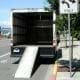Students discover the scientific basis for the use of inclined planes. Using a spring scale, a bag of rocks and an inclined plane, student groups explore how dragging objects up a slope is easier than lifting them straight up into the air.

• Light Plants and Dark Plants, Wet Plants and Dry Ones
Light Plants and Dark Plants, Wet Plants and Dry OnesStudents plant sunflower seeds in plastic cups, and once germinated, expose them to varying light or soil moisture conditions. They measure the seedlings' growth every few days using non-standard measurement (inch cubes).

• Decimals, Fractions & Percentages
Decimals, Fractions & Percentages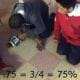Students learn about and practice converting between fractions, decimals and percentages. Using a LEGO® MINDSTORMS® robot and a touch sensor, each group inputs a fraction of its choosing. Team members convert this same fraction into a decimal, and then a percentage via hand calculations, and double ...

• The Beat Goes On
The Beat Goes OnIn this activity, students learn about their heart rate and different ways it can be measured. Students construct a simple measurement device using clay and a toothpick, and then use this device to measure their heart rate under different circumstances (i.e., sitting, standing and jumping)

• Stack It Up!
Stack It Up!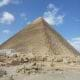Students analyze and begin to design a pyramid. Working in engineering teams, they perform calculations to determine the area of the pyramid base, stone block volumes, and the number of blocks required for their pyramid base.

• Trebuchet Launch
Trebuchet Launch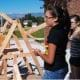Students work as engineers to design and test trebuchets (in this case LEGO® MINDSTORMS® robots) that can launch objects. They determine how far objects travel depending on their weights.

• Timing a Speedbot!
Timing a Speedbot!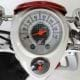Students strengthen their communicate skills about measurements by learning the meaning of base units and derived units, including speed—one of the most common derived units (distance/time). Working in groups, students measure the time for LEGO® MINDSTORMS® robots to move a certain distance.

• The Balancing Act
The Balancing Act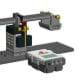Students visualize and interact with concepts already learned, specifically algebraic equations and solving for unknown variables. They construct a balancing seesaw system (LEGO® Balance Scale) made from LEGO MINDSTORMS® parts and digital components to mimic a balancing scale.

• Cleaning the Floor Using a Robot: Intro to Random Numbers
Cleaning the Floor Using a Robot: Intro to Random Numbers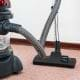Posed with a paradigmatic engineering problem, students consider and explore mathematical algorithms and/or geometric concepts to devise possible solutions. The problem: How should a robotic vacuum move in order to best clean a floor of unknown shape and dimensions?

• Close Enough? Angles & Accuracy of Measurement in Navigation
Close Enough? Angles & Accuracy of Measurement in NavigationStudents use right triangle trigonometry and angle measurements to calculate distances using accuracy and precision. They understand the relationship between triangulation technology and other fields of study (i.e. mathematics).

• Discovering Phi: The Golden Ratio
Discovering Phi: The Golden Ratio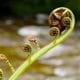Students discover the mathematical constant phi, the golden ratio, through hands-on activities. They measure dimensions of "natural objects"—a star, a nautilus shell and human hand bones—and calculate ratios of the measured values, which are close to phi.

• Forces and Graphing
Forces and GraphingUse this activity to explore forces acting on objects, practice graphing experimental data, and introduce the algebra concepts of slope and intercept of a line. Students learn how to conduct an experiment by applying loads at different locations along the beam, recording the exact position of the ap...

• Applications of Systems of Equations: An Electronic Circuit
Applications of Systems of Equations: An Electronic Circuit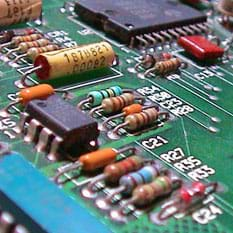Students use Ohm’s law to solve for current values, given a system of linear equations that mathematically models a circuit. From the same schematic, groups create electric circuits using breadboards, resistors and jumper wires. They use voltmeters to measure the current flow across five resistors. ...

• Designing an Elliptical Pool Table
Designing an Elliptical Pool Table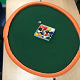Students learn about the mathematical characteristics and reflective property of ellipses by building their own elliptical-shaped pool tables. After a slide presentation introduction to ellipses, student “engineering teams” follow the steps of the engineering design process to develop prototypes, wh...

• Mathematically Designing a Frictional Roller Coaster
Mathematically Designing a Frictional Roller Coaster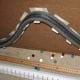Students apply high school differential calculus and physics to design 2D roller coasters in which the friction force is taken into consideration. Student teams first mathematically design the coaster path (using what they learned in the associated lesson) and then use foam pipe wrap insulation mate...

• Volumes of Complex Solids
Volumes of Complex SolidsChallenged with a hypothetical engineering work situation in which they need to figure out the volume and surface area of a nuclear power plant’s cooling tower (a hyperbolic shape), students learn to calculate the volume of complex solids that can be classified as solids of revolution or solids with...

• Can You Hear Me Now?
Can You Hear Me Now?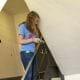Students apply their knowledge of linear regression and design to solve a real-world challenge to create a better packing solution for shipping cell phones. They make composite material packaging containers using cardboard, fabric, plastic, paper and/or rubber bands to create four different-weight p...

TeachEngineering provides lesson plans and activities with math-focused worksheets and assessments to promote mathematical proficiency essential to K-12 youth. Our curriculum stresses the importance of understanding problems, using models and mathematical concepts to solve problems, and developing feasible arguments based on data. We hope you find these math-aligned engineering lessons and activities useful in your classroom!

### Browse All Math-Aligned Engineering Curriculum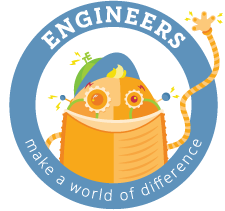Free K-12 standards-aligned STEM curriculum for educators everywhere.
Find more at TeachEngineering.org